# SBI PO Reasoning Questions 2019 (Day-07) High Level-New Pattern

SBI PO 2019 Notification is about to come and it is the most awaited exam among the aspirants. We all know that new pattern questions are introducing every year in the SBI PO exam. Further, the questions are getting tougher and beyond the level of the candidate’s expectations.

Our IBPS Guide is providing High-Level New Pattern Reasoning Ability Questions for SBI PO 2019 so the aspirants can practice it on a daily basis. These questions are framed by our skilled experts after understanding your needs thoroughly. Aspirants can practice these high-level questions daily to familiarize with the exact exam pattern. We wish that your rigorous preparation leads you to a successful target of becoming SBI PO.

#### “Be not afraid of growing slowly; be afraid only of standing still”

[WpProQuiz 4814]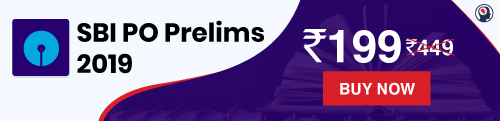### Click Here for SBI PO Pre 2019 High-Quality Mocks Exactly on SBI Standard

(Directions 1–4): A string of numbers is given as input. The further steps given are obtained by applying certain logic. Each step is a resultant of previous step only. Study the following information carefully and answer the questions given below it.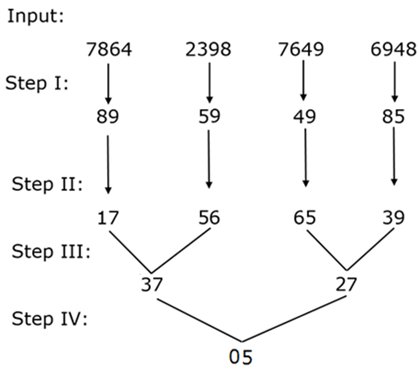As per above applied logic in above steps, find appropriate step for given input: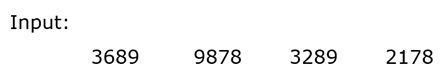1) What will be the difference of highest to lowest number in step II?

a) 21

b) 28

c) 39

d) 32

e) None of these

2) What will be the difference of square of digits of lowest number in step III?

a) 15

b) 55

c) 21

d) 9

e) None of these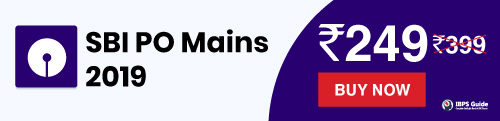3) What will be the sum of digits of all numbers in step I?

a) 54

b) 23

c) 46

d) 28

e) None of these

4) Which of the following is the final output in step IV?

a) 45

b) 36

c) 74

d) 81

e) None of these

(Directions 5–8): Study the following information carefully and answer the questions given below it.

In a certain code language some statements are coded as follow:

“Player Comedy World Victory Final” is coded as “K%W C#F H#N G%P I\$A”.

“Prime Trailer Video Genius Mode” is coded as “[email protected] M\$T E#G P%Q D#Q”.

“Service Token Smart Fear Society” is coded as “B\$G E#V [email protected] E\$A I#P”.

“List Risk Under Valid Project” is coded as “[email protected] O\$V I#T [email protected] H#F”.

5) How “Smart Project” is coded in given code language?

a) I#T [email protected]

b) B\$G H#F

c) E#V O\$V

d) [email protected] E\$A

e) None of these

6) Which of the following statement is coded as “A#V I#P I\$A” in same code language?

a) Mount Victory Token

b) Victory Build Fear

c) Token Count Victory

d) Maker Victory Fear

e) Either A or C

7) How “Genius Mind Power” is coded in given code language?

a) J%T [email protected] M\$T

b) [email protected] J#T P%Q

d) [email protected] P%Q D\$B

e) None of these

8) How “Monthly Policy” is coded in given code language?

a) D%P C\$A

b)B#G D\$T

c) Y%C D%P

d) Either A or C

e) None of these

(Directions 9–10): Study the following information carefully and answer the questions given below it.

[email protected] means – P is north of Q.

P^Q means – P is east of Q.

P%Q means – P is south of Q.

P&Q means – P is west of Q.

If,

[email protected]^Q means P is North-East of Q and so on.

9) In the given expression below what is the position of A with respect to H?

(i) A%B&C

(ii) F&[email protected]

(iii) [email protected]^E

(v) [email protected]&H

(vi) D%^A

(vii) [email protected]^A

(viii) F%&C

a) East

b) North-East

c) South-East

d) North-West

e) Can’t be determined.

10) In the given expression below what is the position of I with respect to B?

(i) A&B%C

(ii) H^G%F

(iii) [email protected]^F

(iv) [email protected]^H

(v) D^C

(vi) F&I

(vii) [email protected]

(viii) [email protected]&F

a) East

b) North-East

c) South-East

d) North-West

e) Can’t be determined.

(Directions 1–4):

We have: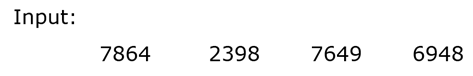Step I: In this step following logic is applied: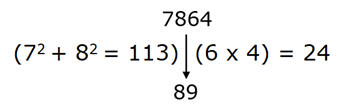Clearly, result in step I can be determined by resultant of above results.

Result = (113 – 24) = 89

Step II: In this step following logic is applied: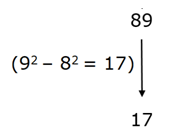Clearly, in step II result can be determined by difference of square of digits.

Step III: In this step following logic is applied: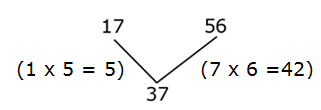Clearly, result in step III can be determined by difference of results.

Result = (42 – 5) = 37

Step IV: In this step following logic is applied: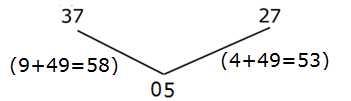Clearly, result in step IV can be determined by difference of results.

Thus, final output is,

Result = (58– 53) = 05

From above logical steps we get following results for given input: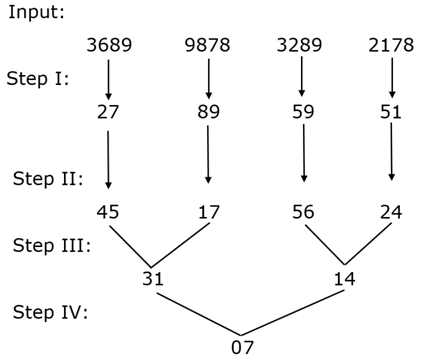Clearly, difference of highest to lowest number = (56 – 17) = 39

Hence, option C is correct choice.

Clearly, lowest number in step III = 14

Thus, required difference = (16 – 1) = 15

Hence, option A is correct choice.

Clearly, required sum = (2 + 7 + 8 + 9 + 5 + 9 + 5 + 1) = 46

Hence, option C is correct choice.

Clearly, desired output in step IV = 07

Hence, option E is correct choice.

(Directions 5–8):

We have:

Player -> G%P

Above statements are coded as follow:

• First letter is the code equivalent to difference of place value of 2nd letter from left and 2nd letter from right.

For E.g.:- L = 12; E = 5;

G = (12 – 5) = 7

• Symbol is code equivalent to number of letters in a word.

For E.g.:- @ = 4; # = 5; % = 6 and \$ = 7.

• Last letter of the code is coded as:-

If number of letters in the given word are even, then (Last letter – 2).

If number of letters in the given word are odd, then (Last letter + 2).

Similarly, we have:

World -> C#F

We have:

Smart -> E#V

Project -> O\$V

Hence, option C is correct choice.

We have:

A#V -> Count/Mount

I#P -> Token

I\$A -> Victory

Hence, option E is correct choice.

Genius -> P%Q

Mind -> [email protected]

Power -> J#T

Hence, option B is correct choice.

We have:

Monthly -> C\$A

Banker -> D%P

Hence, option A is correct choice.

We have:

From above given statements we have: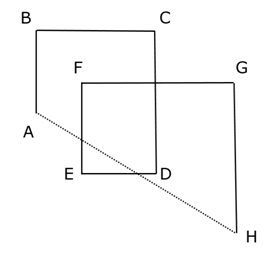Clearly, A is north-west of H.

Hence, option D is correct choice.

We have: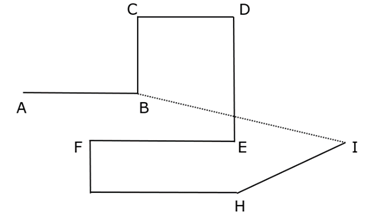Clearly, I is South-East of B.

Hence, option C is correct choice.

### Click Here for SBI PO Pre 2019 High-Quality Mocks Exactly on SBI Standard

Daily Practice Test Schedule | Good Luck

 Topic Daily Publishing Time Daily News Papers & Editorials 8.00 AM Current Affairs Quiz 9.00 AM Current Affairs Quiz (Hindi) 9.30 AM NIACL AO Prelims – Reasoning 10.00 AM NIACL AO Prelims – Reasoning (Hindi) 10.30 AM NIACL AO Prelims – Quantitative Aptitude 11.00 AM NIACL AO Prelims – Quantitative Aptitude (Hindi) 11.30 AM Vocabulary (Based on The Hindu) 12.00 PM NIACL AO Prelims – English Language 1.00 PM SSC Practice Questions (Reasoning/Quantitative aptitude) 2.00 PM IBPS Clerk – GK Questions 3.00 PM SSC Practice Questions (English/General Knowledge) 4.00 PM Daily Current Affairs Updates 5.00 PM SBI PO/IBPS Clerk Mains – Reasoning 6.00 PM SBI PO/IBPS Clerk Mains – Quantitative Aptitude 7.00 PM SBI PO/IBPS Clerk Mains – English Language 8.00 PM